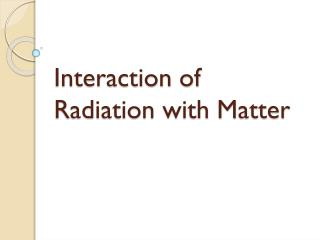DownloadDownload PresentationInteraction of Radiation with Matter

# Interaction of Radiation with Matter

Download Presentation## Interaction of Radiation with Matter

- - - - - - - - - - - - - - - - - - - - - - - - - - - E N D - - - - - - - - - - - - - - - - - - - - - - - - - - -
##### Presentation Transcript

1. Interaction of Radiation with Matter

2. Topics • Radiation intensity • Attenuation • Linear attenuation coefficient • Differential absorption • Half-value layer • Transmission Fraction • X-ray Interaction Mechanism

3. Radiation Intensity • The intensity of radiation is defined as the rate of emitted energy from unit surface area through unit solid angle.

4. Attenuation • The total reduction in the number of x-rays remaining in an x-ray beam after penetration through a given thickness of tissue. • Attenuation is the product of absorption and scattering.

5. Linear Attenuation Coefficient • The attenuation coefficient is a quantity that characterizes how easily a material or medium can be penetrated by a beam of light, sound, particles, or other energy or matter.

6. Differential Absorption • The difference in x-ray interaction. • Increases as the kVp is reduced.

7. Differential absorption and attenuation of the x-ray beam depend on the following factors: • The atomic number (Z) of the atoms in tissue. • The mass density of the atoms in tissue. • The x-ray energy.

8. Half Value Layer (HVL) The Thickness of an absorber needed to reduce the intensity of the x-ray beam into half of its original value.

9. X-ray Interaction Mechanism • Coherent Scattering • Compton Scattering • Photoelectric Effect • Pair Production • Photodisintegration

10. Coherent Scattering • Energies below approximately 10 keV. • Sometimes called classical scattering or Thompson scattering, Rayleigh Scattering. • No ionization.

11. Coherent Scattering

12. Coherent scattering

13. Compton Effect • In the Compton effect, the incident x-ray interacts with an outer shell electron and ejects it from the atom, thereby ionizing the atom. The ejected electron is called a Compton electron or a secondary electron.

14. Compton Effect • The probability of the Compton effect is inversely proportional to x-ray energy (1/E) and independent of atomic number.

15. Compton Effect

16. Compton Effect

17. Photoelectric Effect • Interacts with inner shell electrons • X-rays are absorbed • The electron removed from an atom is called photoelectron.

18. Photoelectric Effect • The probability if the photoelectric effect is inversely proportional to the third power of the x-ray energy (1/E)3. • The probability of photoelectric effect is directly proportional to the third power of the atomic number of the absorbing material (Z3).

19. Photoelectric Effect

20. Photoelectric Effect

21. Atomic Number and K-shell Electron binding energy of radiologically important elements

22. Pair Production • Incident electron interacts with the nuclear field. • The interaction between the x-ray and the nuclear field causes the x-ray to disappear, and in its place, two electrons appear, one positively charged (positron) and one negatively charged (electron).

23. Pair Production

24. Pair Production • Occurs above 1.02 MeV

25. Photodisintegration • Occurs with x-ray energies above 10 MeV. • The nucleus is raised to an excited state and instantly emits a nucleon or other nuclear fragment.

26. Photodisintegration

27. Photodisintegration